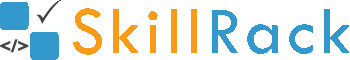Must Solve 100 Programs for Campus Placement Part - 3/104 months ago

1) Odd or Even

The program must print if the given number is Odd or Even.

Example Input/Output 1:
Input:
5

Output:
Odd

Example Input/Output 2:
Input:
102

Output:
Even

Click the link below to solve this program
http://www.skillrack.com/solve/7886

2) Mobile Signal

The program must accept a character representing the mobile signal.
- If the character is E, print 2G
- If the character is H, print 3G
- Else print 4G

Click the link below to solve this program
http://www.skillrack.com/solve/7885

3) Hot Cold or Normal

The program must print Hot if the given temperature value is more than 30, must print Cold if the given value is less than 22. Else the program must print Normal.

Example Input/Output 1:
Input:
25

Output:
Normal

Example Input/Output 2:
Input:
34

Output:
Hot

Example Input/Output 3:
Input:
18

Output:
Cold

Click the link below to solve this program
http://www.skillrack.com/solve/7887

4) Multiply Two Numbers

Accept two numbers and print their product.

Example Input/Output 1:
Input:
10 5

Output:
50

Example Input/Output 2:
Input:
6 5

Output:
30

Click the link below to solve this program
http://www.skillrack.com/solve/7904

5) Two Subjects - pass or fail

The pass mark in a subject is 40. The marks scored in two subjects by a student is passed as the input. If the student has passed in both the subjects, the program must print pass. Else must print fail.

Example Input/Output 1:
Input:
50 60

Output:
pass

Example Input/Output 2:
Input:
34 60

Output:
fail

Click the link below to solve this program
http://www.skillrack.com/solve/7888

6) Atleast One Number 100

The numbers are passed as the input to the program. If at least one of the numbers is equal to 100, the program must print yes. Else the program must print no.

Example Input/Output 1:
Input:
50 100

Output:
yes

Example Input/Output 2:
Input:
34 60

Output:
no

Click the link below to solve this program
http://www.skillrack.com/solve/7889

7) Not Equal to Zero

The program must accept a number and print yes if it is NOT equal to zero. Else it must print no.

Example Input/Output 1:
Input:
0

Output:
no

Example Input/Output 2:
Input:
22

Output:
yes

Click the link below to solve this program
http://www.skillrack.com/solve/7893

8) Division - Two Values

The program must accept two numbers and print the value (up to two decimal places) when they are divided.

Example Input/Output 1:
Input:
10 4

Output:
2.50

Example Input/Output 2:
Input:
20 3

Output:
6.67

Click the link below to solve this program
http://www.skillrack.com/solve/7891

9) Canteen Expense

A student has Rs.100 in hand. He buys snacks for X rupees from the canteen. The program must print the amount remaining with the student.

Note: The program must accept the value of X as the input.

Example Input/Output 1:
Input:
60

Output:
40

Example Input/Output 2:
Input:
75

Output:
25

Click the link below to solve this program
http://www.skillrack.com/solve/7902

10) Average - Two Numbers

The program must accept two numbers and print their average (up to two decimal places).

Example Input/Output 1:
Input:
10 4

Output:
7.00

Example Input/Output 2:
Input:
20 3

Output:
11.50

Click the link below to solve this program
http://www.skillrack.com/solve/7892